# 安装部署环境

In this passage I
want to recode the content of have to install the purosim in ubuntu 16.04 for
remeber.

use this web to

`bash</p><p style="font-family:Calibri;font-size:0.917rem;">git clone <a href="https://github.com/ccappelle/pyrosim.git">https://github.com/ccappelle/pyrosim.git</a></p><p style="font-family:Calibri;font-size:0.917rem;">`

Follow the github https://github.com/ccappelle/pyrosim to install the siftware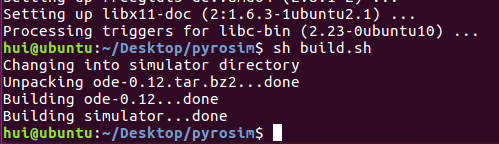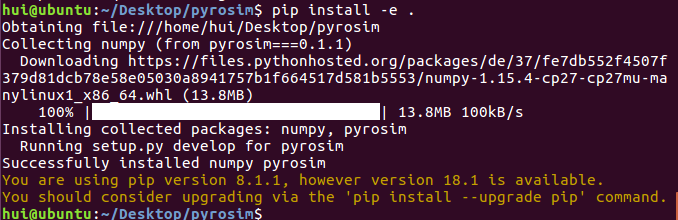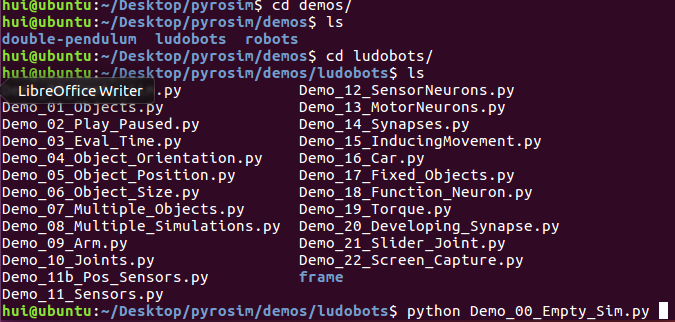Now We can
start the first Demo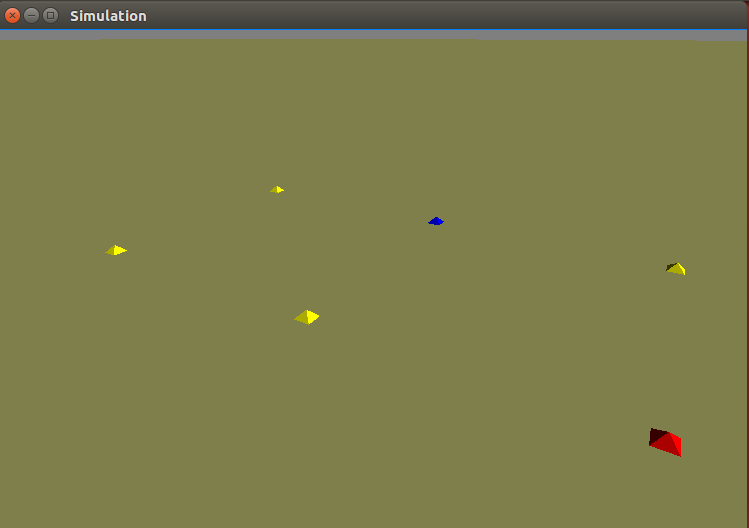This should bring up an
OpenGL window displaying an empty, virtual world. The window will close on its
own after a few seconds.

Notes

have pyrosim installed and want the latest update from the repository, change
into the modules root directory (contains setup.py) and pull

\$ git pull

The C++ code is
likely to have changed so you have to recompile it by going int the simulator
directory and typing make

\$ cd
pyrosim/simulator

\$ make

The simulator
directory contains all of the C++ code used in pyrosim.

Next steps.

Now you can start
making robots here,
starting at step #3.

In this part

# 3

We should
to make sure that

we have
the env of the numpy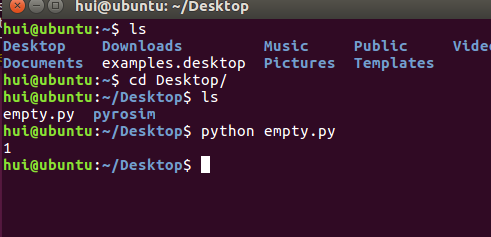We finish
the env.

Till now
we finsh the env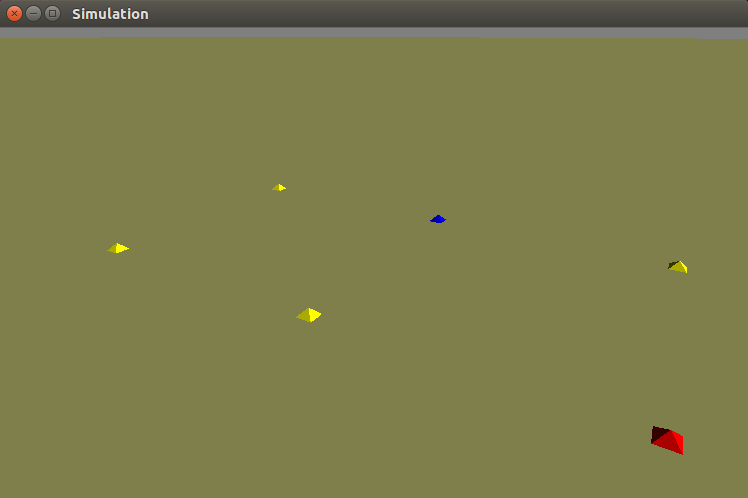Next We
start to do the assgn 2

Follow
the title do

When the
code show as the follow we will see the
1 & 0.1 tools pause in the
Simulation.

Object.py

`python</p><p style="font-family:Calibri;font-size:0.917rem;"> </p><p style="font-family:Calibri;font-size:0.917rem;">`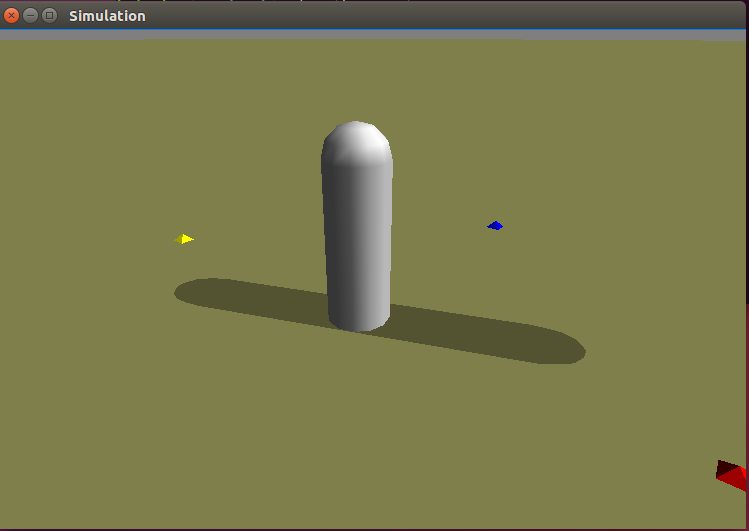When we
come to there ,we can use the ctrl + P to release the pause .And we can use the
ctrl + o to view the simulation motion step by step.

We can
use the mouse to adjust the position of the view we see. The left button is
change the view in 3D view and the right view change the view of far or near.

When we
change the original date like the follow

sim.send_cylinder( x=0
, y=0 , z=0.6 , length=1.0 , radius=0.1 )

We will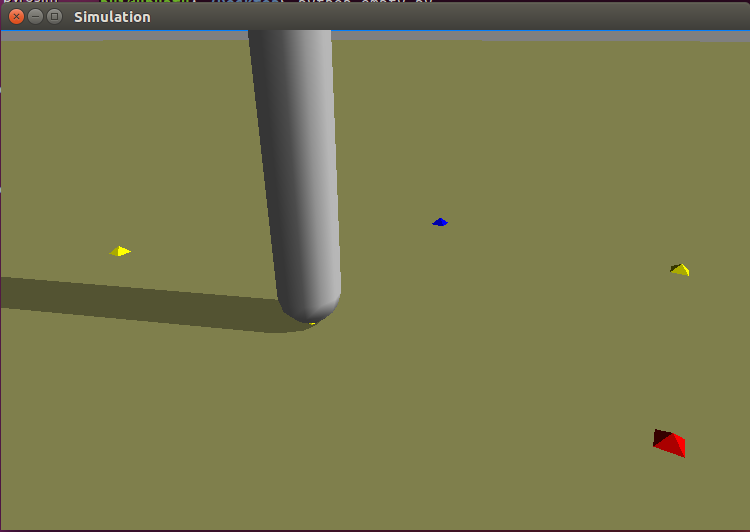Why the data is 0.6 because the half of the cylinder is 0.5 and the
radius is 0.1. so we should make the
z=0.6 ,which can make the cylinder and the half ball out of the coordinate(

2019-1-14 3:43 the process of this task has to the 15
prolem.

When we
change the location of the cylinder at this code

sim.send_cylinder( x=0
, y=0 , z=1.5 , length=1.0 , radius=0.1 )

We will see the situation about
the cylinder .

 And we can tell the simulator to rrun for 1000 times steps.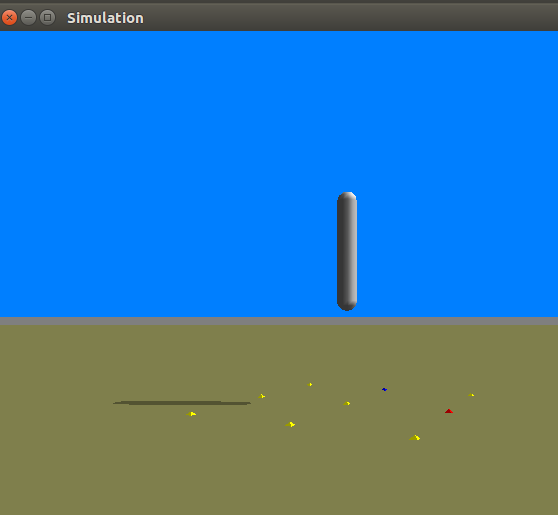import pyrosim

sim=pyrosim.Simulator(play_paused=True,eval_time=1000)

print(whiteObject)

redObject=sim.send_cylinder(x=-0.2,y=0,z=0.6)

print(redObject)

sim.start()

In the
command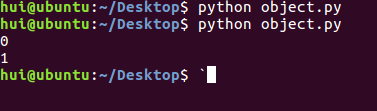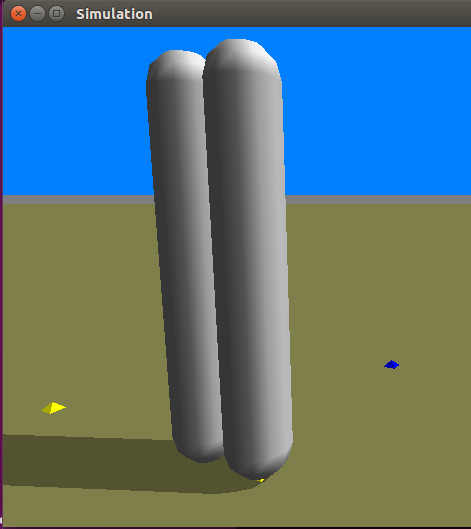Change
the color of the next .

redObject=sim.send_cylinder(x=-0.2,y=0,z=0.6,r=1,
g=0, b=0)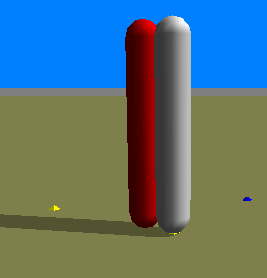rolation vectoylinder

Change
the red_cu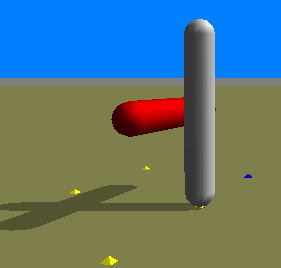Now I
push myself to the next question

In order
to get the f pic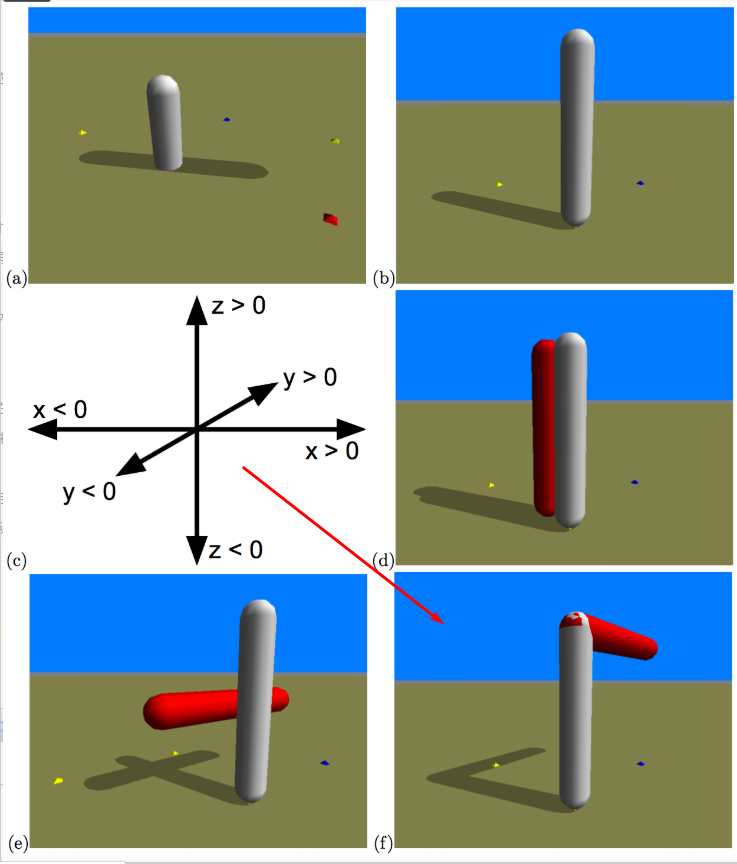This is
so easy my code is in the next lineSO I finish the part of pyrosim/objects

<wiz_tmp_tag id=”wiz-table-range-border” contenteditable=”false” style=”display: none;”></wiz_tmp_tag>